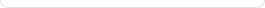# Wolfram webMathematica

What Is webMathematica?

webMathematica adds interactive calculations and visualization to websites by integrating Mathematica with the latest web server technology.## How is webMathematica different from Mathematica?

webMathematica and Mathematica have the same underlying engine, but they provide fundamentally different user interfaces and are aimed at different types of users.

webMathematica offers access to specific Mathematica applications through a web browser or other web clients. The standard interface provided requires little training to use effectively. In most cases, users neither have to be familiar with Mathematica nor need to know they are using Mathematica.

Similarly, webMathematica developers need only a basic knowledge of HTML and Mathematica to create complete, full-featured websites. Other technical programs require Java programming skills and only allow creation of small applets. Also, because webMathematica can access the full range of Mathematica's built-in computational abilities, developers don't need to work with extra code libraries.

In some sense, Mathematica is a development environment for webMathematica sites. As an example, you can work on code in Mathematica that models some physical process—code that can then be placed into a webMathematicasite to enable others to run the model and use its results for their regular work.